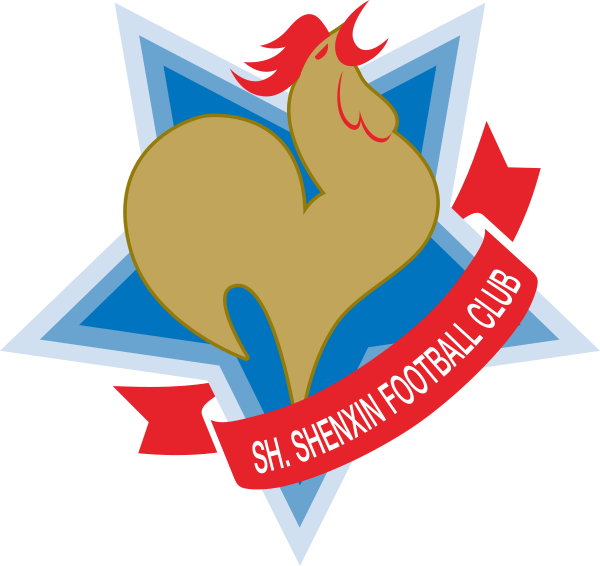# some category theory 1

The main reference: Methods of Homological Algebra.

1. Suppose $\mathcal{C}$ is a category, $\mathcal{C}^\circ$ is its dual category. Thus any contravariant functor $\varphi:\mathcal{C}\to D$ is a (covariant) functor $\varphi:\mathcal{C}^\circ\to \mathcal{D}$ .

2. Suppose $\mathcal{C}$ and $\mathcal{D}$ are categories. We can construct a category of functors form $\mathcal{C}$ to $\mathcal{D}$ by the follow steps. Firstly, the objects in $\textit{Funct}(\mathcal{C},\mathcal{D})$ are functors form $\mathcal{C}$ to $\mathcal{D}$ . Then, a morphism $f$ of functors from $F$ to $G$ (notation $f:F\to G$ ) is a family of morphisms in $\mathcal{D}$ that $f(X):F(X)\to G(X),$ one for each $X\in \mathcal{C}$ , satisfying the following condition: for all morphism $\varphi$ in $\mathcal{C}$ the following diagram $\require{AMScd} \begin{CD} F(X) @>f(X)>> G(X)\\ @V{F(\varphi)}VV @VV{G(\varphi)}V \\ F(Y) @>f(Y)>> G(Y) \end{CD}$ is commutative. If $F$ is isomorphic to $G$ (notation $F\cong G$ ), we should have morphisms $f$ and $g$ such that $f(X)\circ g(X)=\operatorname{id}_{G(X)}$ and $g(X)\circ f(X)=\operatorname{id}_{F(X)}$ for all $X\in \mathcal{C}$ .

3. Suppose $\hat{\mathcal{C}}=\textit{Funct}(\mathcal{C}^\circ,\textit{Set})$ , $\forall X\in \mathcal{C}$ , we can associate a $\hat{X}\in \hat{\mathcal{C}}$ by $\hat{X}(Y)=\operatorname{Hom}_\mathcal{C}(Y,X)$ and $\hat{Z}(\varphi)f=f\circ \varphi$ for $\varphi \in \operatorname{Hom}_\mathcal{C}(Y,X)$ and $f\in \operatorname{Hom}_\mathcal{C}(X,Z)$ . Then $\hat{Z}(\varphi):\hat{Z}(X)\to \hat{Z}(Y)$ .

Proposition 1: Suppose $X$ is a set in the category Set, it can be identified with the set $\hat{X}(e)=\operatorname{Hom}_{\textit{Set}}(e,X)$ , where $e$ is a one-point set.

Proof. Obviously.

Indeed, in an arbitrary category $\mathcal{C}$ an analogue of one-point set does not necessarily exist. However, by considering $\operatorname{Hom}_{\mathcal{C}}(Y,X)$ for all $Y\in \mathcal{C}$ simultaneously, we can recover complete information about an object $X\in \mathcal{C}$ . This is the idea of representation theory.

Definition 1: A functor $F\in \hat{\mathcal{C}}$ is said to be representable if $F\cong \hat{X}$ for some $X\in \mathcal{C}$ . One says also that the object $X$ represents the functor $F$ .

Then

Theorem 1: $\operatorname{Hom}_\mathcal{C} (X,Y)\cong \operatorname{Hom}_{\hat{\mathcal{C}}} (\hat{X},\hat{Y})$ .

Proof. Let $\varphi:X\to Y$ be a morphism in $\mathcal{C}$ . We associate with $\varphi$ the morphism of functors $\hat{\varphi}:\hat{X}\to \hat{Y}$ by $\hat{\varphi}(Z):\theta\mapsto\varphi\circ\theta\in \hat{Y}(Z),$ where $\theta\in \hat{X}(Z)$ and $X$ , $Y$ , $Z\in \mathcal{C}$ . It is clear that $\hat{\varphi}\circ \hat{\phi}=\widehat{\varphi\circ\phi}$ . Conversely, suppose $f\in \operatorname{Hom}_{\hat{\mathcal{C}}} (\hat{X},\hat{Y})$ , define map $i:\operatorname{Hom}_{\hat{\mathcal{C}}} (\hat{X},\hat{Y})\to \operatorname{Hom}_\mathcal{C} (X,Y)$ by $i:f\mapsto f(X)(\operatorname{id}_X),$ where $\operatorname{id}_X\in \operatorname{Hom}_\mathcal{C}(X,X)=\hat{X}(X)$ and $f(\operatorname{id}_X)\in \hat{Y}(X)$ . Then $i(\hat{\varphi})=\hat{\varphi}(X)(\operatorname{id}_X)=\varphi\circ \operatorname{id}_X=\varphi.$ On the other hand, we should show $\widehat{i(f)}=f$ when $f\in \operatorname{Hom}_{\hat{\mathcal{C}}} (\hat{X},\hat{Y})$ , and it's equivalent to show $\widehat{i(f)}(Z)=f(Z)$ for all $Z\in\mathcal{C}$ . Now suppose a morphism $\varphi:Z\to X$ , $i(f)\circ\varphi=\widehat{i(f)}(Z)(\varphi)=f(Z)(\varphi).$ Using the commutativity of the diagram, $\require{AMScd} \begin{CD} \hat{X}(X) @>f(X)>> \hat{Y}(X)\\ @V{\hat{X}(\varphi)}VV @VV{\hat{Y}(\varphi)}V \\ \hat{X}(Z) @>f(Z)>> \hat{Y}(Z) \end{CD}$ then $f(Z)\circ\hat{X}(\varphi)(\operatorname{id}_X)=\hat{Y}(\varphi)\circ f(X)(\operatorname{id}_X)=\hat{Y}(\varphi)(i(f))=i(f)\circ \varphi$ and $f(Z)\circ\hat{X}(\varphi)(\operatorname{id}_X)=f(Z)(\operatorname{id}_X\circ\varphi)=f(Z)(\varphi).$ Thus $i$ is an isomorphism that $i:\operatorname{Hom}_{\hat{\mathcal{C}}} (\hat{X},\hat{Y})\cong \operatorname{Hom}_\mathcal{C} (X,Y)$ .

If $X$ represents the functor $F$ , $\operatorname{Hom}_{\hat{\mathcal{C}}}(\hat{Y},F)\cong \operatorname{Hom}_{\hat{\mathcal{C}}}(\hat{Y},\hat{X})\cong\operatorname{Hom}_\mathcal{C}(Y,X)$ . If $Y$ also represents $F$ , then there exists an isomorphism $\varphi:\hat{Y}\cong F$ , then $i(\varphi)$ is the according isomorphism between $X$ and $Y$ . Thus, the representing object of a representable functor is defined uniquely up to a isomorphism.

Definition 2: Suppose $X$ , $Y\in\mathcal{C}$ , the direct product $X\times Y$ is (upto an isomorphism) the object $Z$ representing the functor (if such functor is representable, or if such $Z$ exists) $W\mapsto \hat{X}(W)\times\hat{Y}(W),$ where $\hat{X}(W)\times\hat{Y}(W)$ is the direct product of Set which has been constructed directly.

Definition 3: Suppose $X$ , $Y$ , $S\in\textit{Set}$ , $f:X\to S$ and $g:Y\to S$ , the pullback of $f$ and $g$ or the fibre product of $X$ and $Y$ is $X\times_S Y=\{(x,y)\in X\times Y:f(x)=g(y)\}.$ In a general category $\mathcal{C}$ , $X\times_SY$ is defined as the object $Z$ representing the functor $W\mapsto \hat{X}(W)\times_{\hat{S}(W)}\hat{Y}(W).$ When $f=g$ are constant morphisms, $X\times_S Y\cong X\times Y$ . When $f$ and $g$ are embedding, $X\times_S Y\cong X\cap Y$ .

The universal property can be directly vertified. Since $Z$ represents the functor $W\mapsto \hat{X}(W)\times\hat{Y}(W)$ , then $\hat{Z}(W)=\hat{X}(W)\times\hat{Y}(W)$ . Let $W=Z$ , the image of $\operatorname{id}_Z\in \hat{Z}(Z)$ is that $\operatorname{id}_Z=(\pi_X,\pi_Y)$ , where $\pi_X\in \hat{Y}(Z)$ and $\pi_Y\in \hat{Y}(Z)$ . This can be writen as $X\xleftarrow{\pi_X} Z \xrightarrow{\pi_Y}Y$

Now suppose $p_X:W\to X$ and $p_Y:W\to Y$ , then there exist $\eta=(p_X,p_Y)\in \hat{X}(W)\times\hat{Y}(W)= \hat{Z}(W)$ . The last thing we should to show is that $p_X=\pi_X\circ \eta$ and $p_Y=\pi_Y\circ \eta$ . Indeed, for all $z\in Z$ , $(\pi_X,\pi_Y)((p_X,p_Y)(z))=\operatorname{id}_Z((p_X,p_Y)(z))=(p_X,p_Y)(z).$ The uniqueness of $X\times Y$ up to an isomorphism can be drived from the above theorem or universal property directly.

Because of some psychological reasons, we usually use a commutative diagram to visualize the universal property described above: $\begin{xy} \xymatrix{ &A\ar[dl]\ar[dr]\ar@{-->}[d]&\\ X&X\times Y\ar[l]\ar[r]&Y\\ } \end{xy}$ Similarly, the universal property of fibre product can be written as a commutative diagram: $\begin{xy} \xymatrix{ U \ar@/_/[ddr]_y \ar@{.>}[dr]|{\langle x,y \rangle} \ar@/^/[drr]^x \\ & X \times_Z Y \ar[d]^q \ar[r]_p & X \ar[d]_f \\ & Y \ar[r]^g & Z } \end{xy}$

We fix a category $\mathcal{J}$ , and define the diagonal functor $\Delta:\mathcal{C}\to \textit{Funct}(\mathcal{J},\mathcal{C})$ as follow:

• On objects: $\Delta X$ is the set of constant functors with the value $X$ . In other words, $\Delta X(j)=X$ for all $j\in\mathcal{J}$ , $\Delta X(\varphi)=\operatorname{id}_X$ for all morphism $\varphi$ in $\mathcal{J}$ .
• On morphisms: Suppose $\psi:X\to Y$ is a morphism in $\mathcal{C}$ , $\Delta\psi:\Delta X\to \Delta Y$ is defined as follows: $\Delta\psi(j):X=\Delta X(j)\to Y=\Delta Y(j)$ for all $j\in \mathcal{J}$ .

It is clear that $\Delta(\varphi\circ\psi)=\Delta\varphi\circ\Delta\psi$ , so $\Delta$ is indeed a functor.

Definition 4: Suppose $F:\mathcal{J}\to \mathcal{C}$ is a functor, the (projective or inverse) limit of $F$ in the category $\mathcal{C}$ according to $\mathcal{J}$ is an object $X\in\mathcal{C}$ representing the functor $Y\mapsto \operatorname{Hom}_{\textit{Funct}(\mathcal{J},\mathcal{C})}(\Delta Y,F):\mathcal{C}^\circ\to \textit{Set}.$ The limit of $F$ is denoted by $X=\underleftarrow{\lim} F$ .